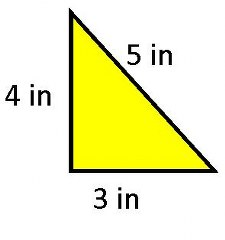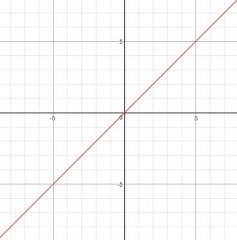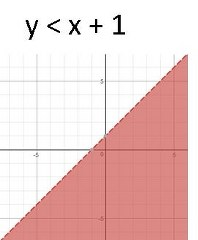# What is a Boundary Line in Math? - Definition & Examples

Instructor: Maria Airth

Maria has a Doctorate of Education and over 20 years of experience teaching psychology and math related courses at the university level.

Boundary lines in math can relate to shapes or areas on an inequality graph. This lesson reviews the definition of boundary lines and gives examples of boundary lines for both shapes and inequality graphs.

## What Is A Boundary?

Let's pretend you just got a new dog. You have a big yard, but no fence. You want to teach the dog how to stay in your yard and not run out in the street or into your neighbor's property. How do you do that? You would probably put the dog on a leash and walk him around the edge of the property. Every day, you would walk the dog around the edge of the property multiple times teaching him how far he is allowed to go.

Boundary lines in math are the same, they identify the outer edge (or outline) of a shape or area. This could be a geometric shape or an inequality graph. Each of these mathematical concepts includes a boundary line. Let's look at each in turn to get a better understanding of boundary lines and how they work.

## Geometric Boundary Lines

When you did boundary training with your dog, you walked around the edge of your property line. If you were to look down at your property from a bird's eye view, you would see a geometric shape. Many properties are rectangular, but not all are. No matter the shape of your property, the boundary line of your property will create a geometric shape.

In math, we use the term perimeter to indicate the distance around the outer edge of a shape. In essence, when you do boundary training with your dog, you are walking the perimeter of your property. To find the perimeter (boundary line) of a shape, just add up the length of all the sides.

Perimeter = sum of sides

### Perimeter Example 1

Ben's rectangular-shaped yard is 500ft across and 700ft deep. What is the measure of the boundary line for this yard?

Solution: Remembering that boundary line and perimeter are the same thing, we find the perimeter by adding up all the sides. P = 500 + 500 + 700 + 700 = 2400 ft

{Hint: Remember that a rectangle has four sides and opposite sides are equal; that's why we have two 500's and two 700's.}

### Perimeter Example 2

What is the perimeter of this shape?Solution: P = sum of sides = 4 + 5 + 3 = 12 in

Notice that the black line is the boundary line, or perimeter, of this yellow triangle.

## Inequality Boundary Lines

When you graph an equation, you get a line. Right? Yes, if it is a true equation with an equal sign the graph will result in a line of some sort. For example, the equation y = x will be a straight line stretching from the bottom right to the top left corners of the graph, like this:But, when you have an inequality the two sides of the mathematical sentence are not always the same value and the graph often ends up looking like a line with a shaded area. The graph of the line, as if it is an equation, is the boundary line for the inequality.

### Inequality Example 1

Look at this graph:To unlock this lesson you must be a Study.com Member.

### Register to view this lesson

Are you a student or a teacher?

#### See for yourself why 30 million people use Study.com

##### Become a Study.com member and start learning now.
Back
What teachers are saying about Study.com

### Earning College Credit

Did you know… We have over 200 college courses that prepare you to earn credit by exam that is accepted by over 1,500 colleges and universities. You can test out of the first two years of college and save thousands off your degree. Anyone can earn credit-by-exam regardless of age or education level.